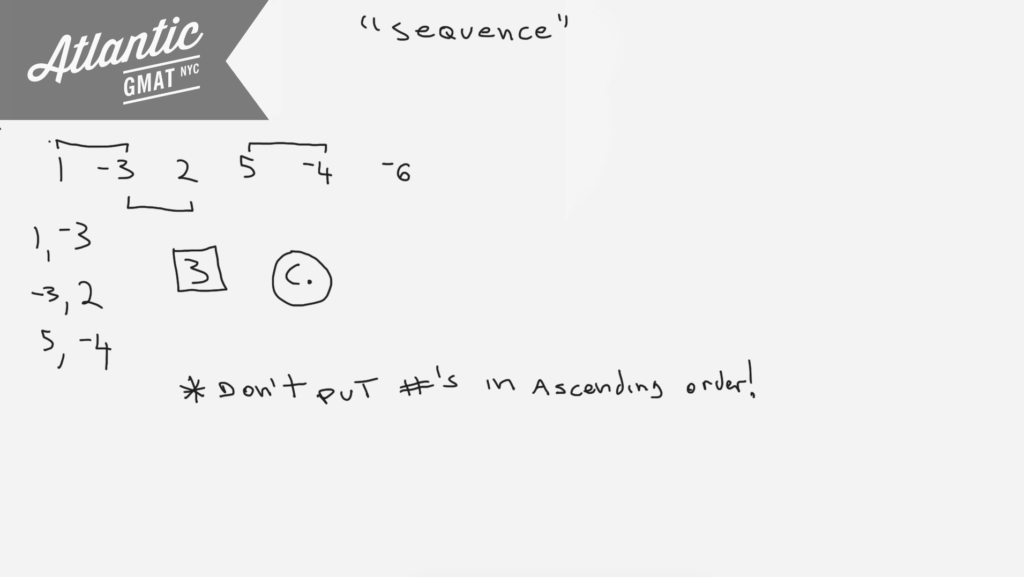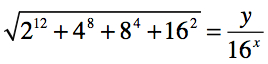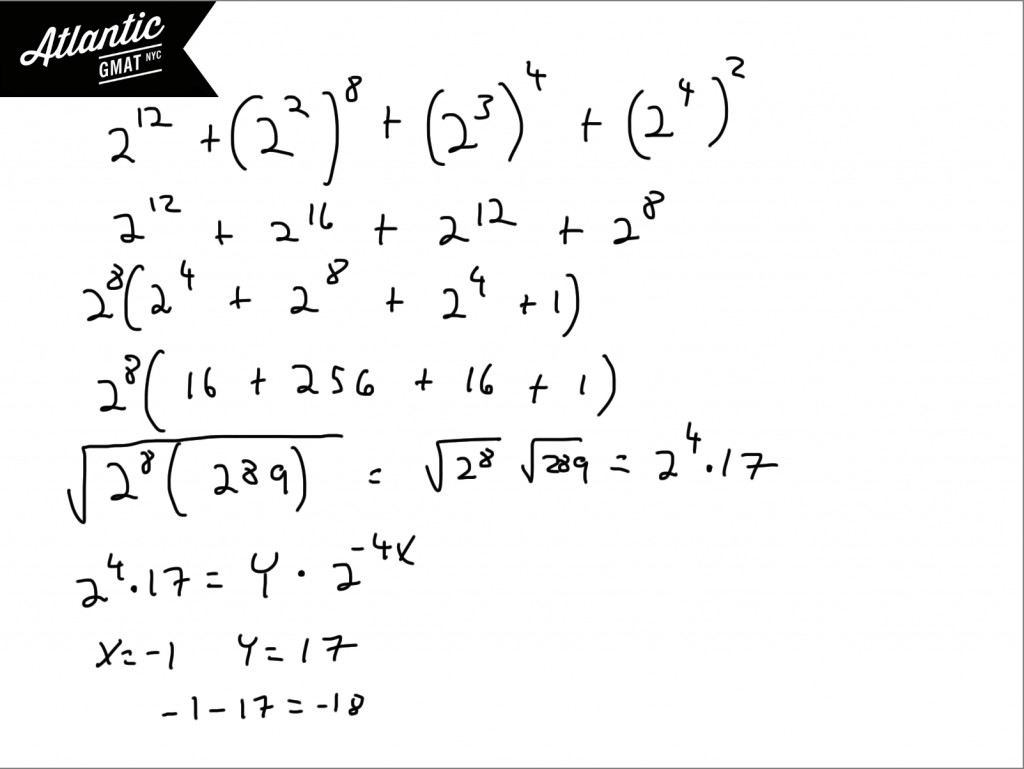Private GMAT Tutoring in NYC & Online

For a finite sequence of non zero numbers, the number of variations in sign is defined as the number of pairs of consecutive terms of the sequence for which the product of the two consecutive terms is negative. What is the number of variations in sign for the sequence 1, -3, 2, 5, -4, -6 ?

(A) 1
(B) 2
(C) 3
(D) 4
(E) 5For a certain race, 3 teams were allowed to enter 3 members each. A team earned 6 – n points whenever one of its members finished in nth place, where 1 ≤ n ≤ 5. There were no ties, disqualifications, or withdrawals. If no team earned more than 6 points, what is the least possible score a team could have earned?

(A) 0
(B) 1
(C) 2
(D) 3
(E) 4

Here's another one from the GMAT Prep Software free tests 1 and 2 so better to wait on this explanation unless you've done those tests already. This is another GMAT puzzle that relies on you being organized. You basically need to make a list of the possibilities keeping track of the constraints with the goal of maximizing the points for two of the teams so the third team has the least possible score. In terms of the zoomed out approach this question reminds of this is other GMAT puzzle: The number 75 can be written as the sum of the squares of 3 different positive integersIf M = √4 + 4^1/3 + 4^1/4, then the value of M is:

(A) Less than 3
(B) Equal to 3
(C) Between 3 and 4
(D) Equal to 4
(E) Greater than 4

If n denotes a number to the left of 0 on the number line such that the square of n is less than 1/100, then the reciprocal of n must be

(A) Less than -10
(B) Between -1 and -1/10
(C) Between -1/10 and 0
(D) Between 0 and 1/10
(E) Greater than 10

# GMAT Question of the Day - Problem Solving - Exponents/Puzzle 3If x and y are both integers, what is the value of x-y?

A. 12

B. 8

C. 0

D. -10

E. -18

## GMAT Question of the Day Solution

When you see a GMAT Exponents question a few things should be coming to mind: The bases, factoring, and special quadratics. In this question you should notice that all of the bases are powers of 2. You might consider getting all of the bases to be the same so that you can do some factoring. Ultimately you want to eliminate the addition so that you can deal with the square root. There's a pretty good chance that something nice is going to happen with the square root considering that all of the answers are integers.

Once you get all of the bases the same you can factor out the smallest one, 2^8. Then all of the exponents in the parenthesis can be resolved. The parenthesis is equal to 289 which is a perfect square. 2^8 is also a perfect square as is any integer raised to an even power. At this point you can deduce that y must be equal to 17 and x to -1. So -1 - 17 = -18. These questions are considered tough but should be your bread and butter!# CONTACT

Atlantic GMAT

405 East 51st St.

NY, NY 10022

(347) 669-3545

info@AtlanticGMAT.com